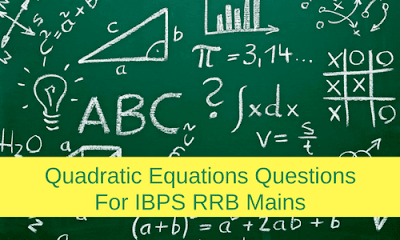New Students Offer - Use Code HELLO

# Quadratic Equations Questions For IBPS RRB Mains1. Direction: In the following question two equations (A) and (B) given. You have to solve both:
1.2.1. If2. If3. If4. If5. If2. Direction: In the following question two equations (A) and (B) given. You have to solve both:
1.2.1. If2. If3. If4. If5. If3. Direction: In the following question two equations (A) and (B) given. You have to solve both:
1.2.1. If2. If3. If4. If5. If4. Direction: In the following question two equations (A) and (B) given. You have to solve both:
1.2.1. If2. If3. If4. If5. If5. Direction: In the following question two equations (A) and (B) given. You have to solve both:
1.2.1. If2. If3. If4. If5. If6. Direction: In the following question two equations (A) and (B) given. You have to solve both:
1.2.1. If2. If3. If4. If5. If7. Direction: In the following question two equations (A) and (B) given. You have to solve both:
1.2.1. If2. If3. If4. If5. If8. Direction: In the following question two equations (A) and (B) given. You have to solve both:
1.2.1. If2. If3. If4. If5. If9. Direction: In the following question two equations (A) and (B) given. You have to solve both:
1.2.1. If2. If3. If4. If5. If10. Direction: In the following question two equations (A) and (B) given. You have to solve both:
1.2.1. If2. If3. If4. If5. If#### 1. Ans. D.

Solution#### 2. Ans. D.

Solution#### 3. Ans. A.

Solution#### 4. Ans. D.

Solution#### 5. Ans A

Solution:#### 6. Ans. E.

Solution#### 7. Ans. B.

Solution:#### 8. Ans. A.

Solution:#### 9. Ans. D.

Solution:#### 10. Ans. D.

Solution